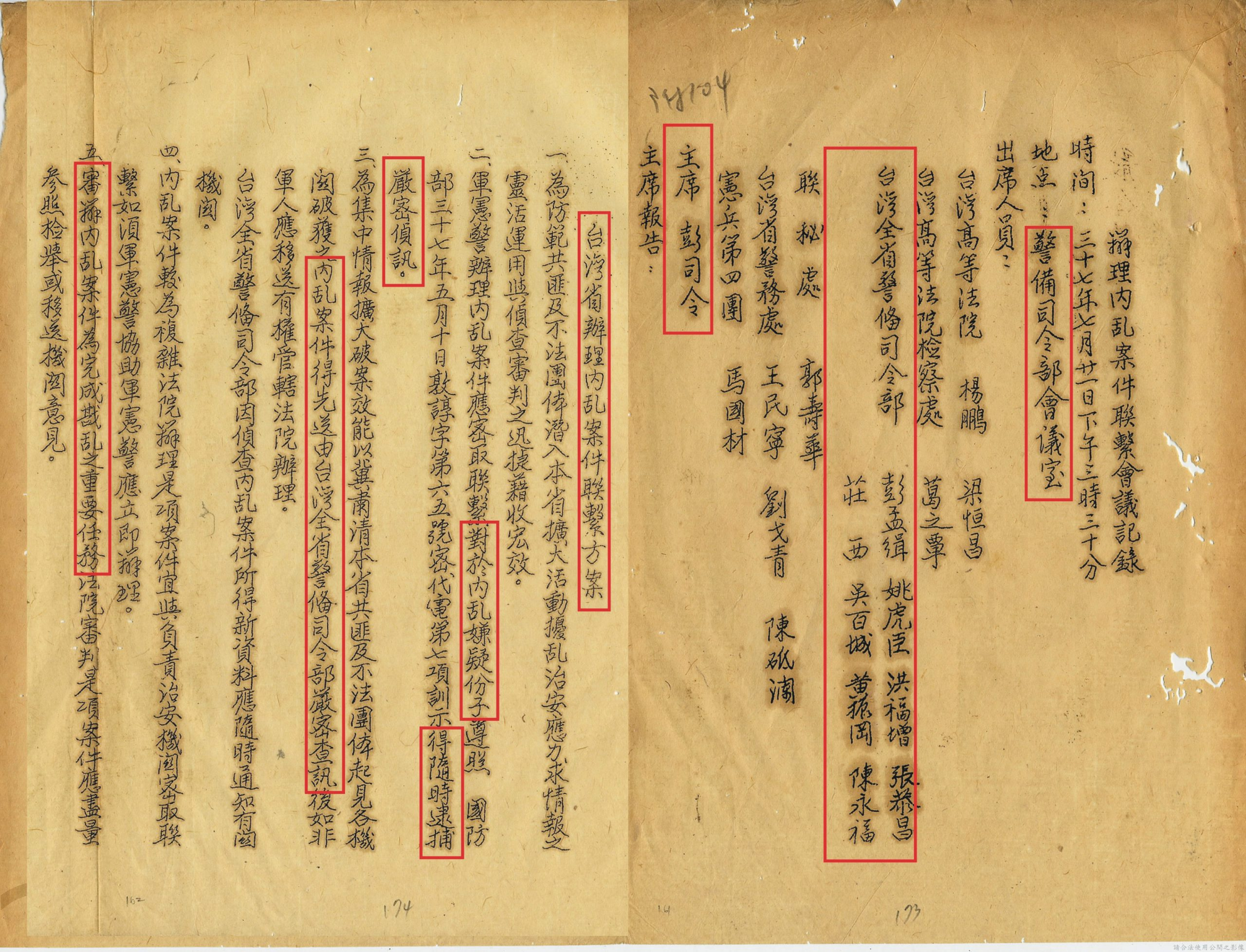# 被遺忘的…警備總部（下）警備總部迫害人權血淚斑斑【註十】《止痛療傷：白崇禧將軍與二二八》，白先勇、廖彥博合著，二○一四年三月十日，時報出版社。

【註十一】檔案管理局，檔號：B5018230601=0036=109.3=4010=002=002=0020

【註十二】檔案管理局，檔號：A305440000C=0040=273.4=1=0001=virtual013

【註十三】檔案管理局，檔號：A305440000C=0040=273.4=1=0001=virtual004=0014

【註十四】檔案管理局，檔號：A305440000C/0056/1571.72/4040.5

【註十五】引自《財團法人台灣民主基金會》二○○九年舉辦的「戒嚴時期白色恐怖與轉型正義」研討會論文集，張炎憲撰寫的〈導言：白色恐怖與轉型正義〉一文。

【註十六】檔案管理局，檔號：A305440000C=0069=1571=6=1=001=0382